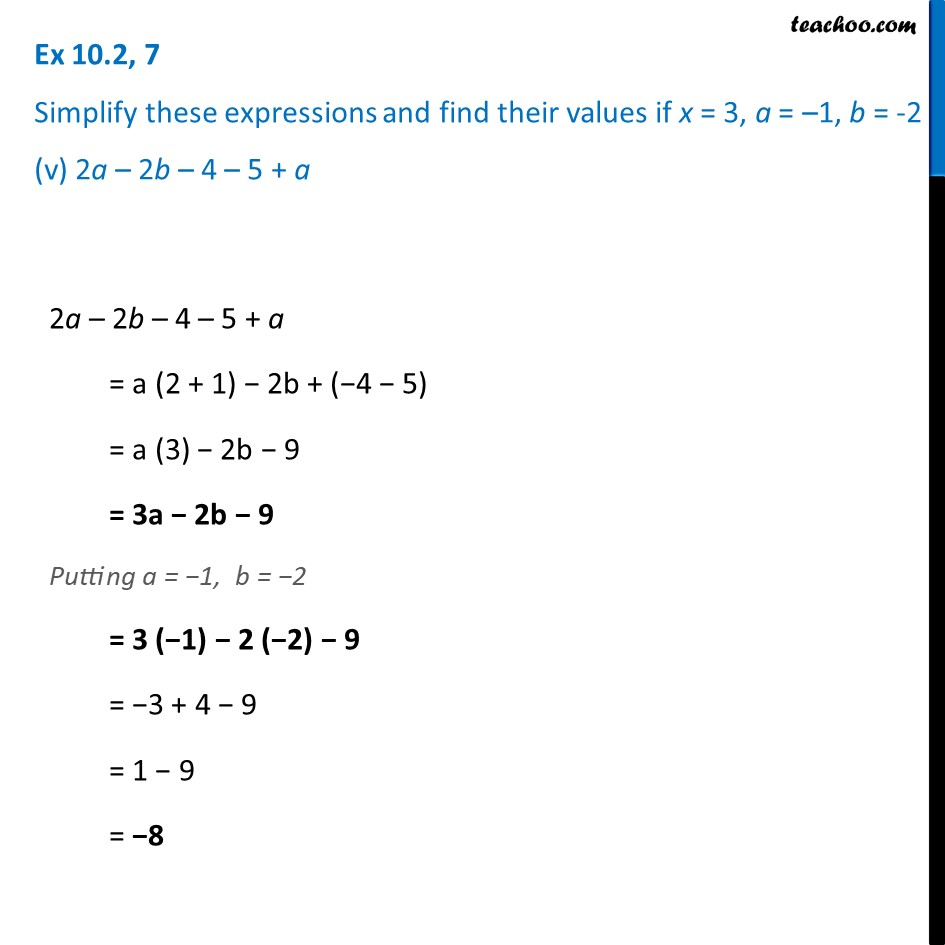Ex 10.2

Chapter 10 Class 7 Algebraic Expressions
Serial order wiseLearn in your speed, with individual attention - Teachoo Maths 1-on-1 Class

### Transcript

Ex 10.2, 7 Simplify these expressions and find their values if x = 3, a = –1, b = -2 (v) 2a – 2b – 4 – 5 + a2a – 2b – 4 – 5 + a = a (2 + 1) − 2b + (−4 − 5) = a (3) − 2b − 9 = 3a − 2b − 9 Putting a = −1, b = −2 = 3 (−1) − 2 (−2) − 9 = −3 + 4 − 9 = 1 − 9 = −8